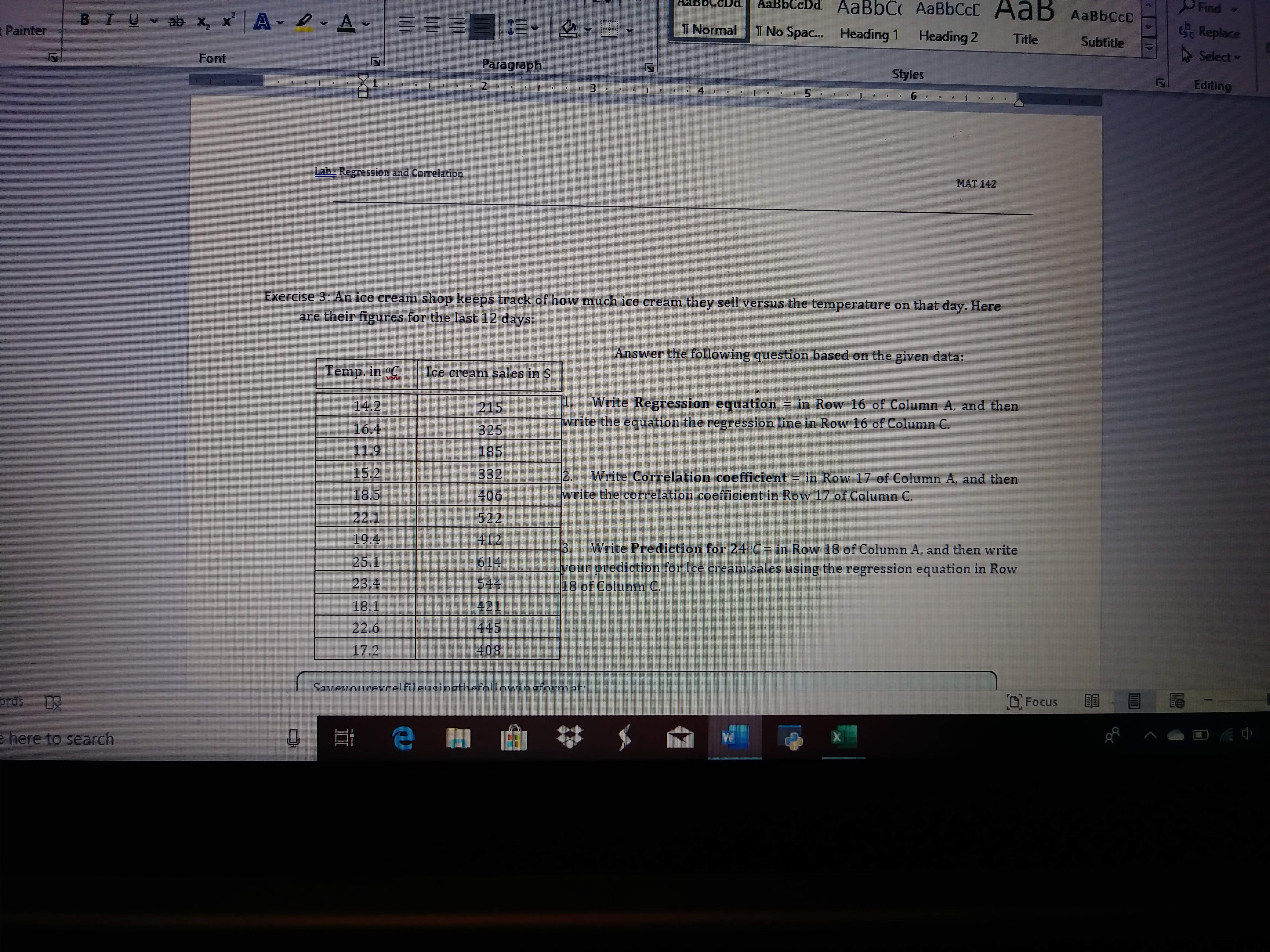# AaBbC AABBCCCAaBbCcDdFindAaB AaBbCcDBIU ab x, x AA -T NormalReplaceT No Spa..PainterHeading 1Heading 2TitleSubtitleA Select -FontParagraphStylesEditing3.5.Lab Regression and CorrelationMAT 142Exercise 3: An ice cream shop keeps track of how much ice cream they sell versus the temperature on that day. Hereare their figures for the last 12 days:Answer the following question based on the given data:Temp. in CIce cream sales in S1.Write Regression equation in Row 16 of Column A, and thenwrite the equation the regression line in Row 16 of Column C.14.221516.432511.918515.233212.Write Correlation coefficient = in Row 17 of Column A, and thenwrite the correlation coefficient in Row 17 of Column C.18.540622.152219.44123.Write Prediction for 24°C = in Row 18 of Column A, and then write25.1614your prediction for Ice cream sales using the18 of Column C.regression equation in Row23.454418.142144522.640817.2Savevourerelfileusinothefnllnuinoform atO Focusordse here to search

Question
45 views

Need help with Question 1help_outlineImage TranscriptioncloseAaBbC AABBCCC AaBbC cDd Find AaB AaBbCcD BIU ab x, x A A - T Normal Replace T No Spa.. Painter Heading 1 Heading 2 Title Subtitle A Select - Font Paragraph Styles Editing 3. 5. Lab Regression and Correlation MAT 142 Exercise 3: An ice cream shop keeps track of how much ice cream they sell versus the temperature on that day. Here are their figures for the last 12 days: Answer the following question based on the given data: Temp. in C Ice cream sales in S 1. Write Regression equation in Row 16 of Column A, and then write the equation the regression line in Row 16 of Column C. 14.2 215 16.4 325 11.9 185 15.2 332 12. Write Correlation coefficient = in Row 17 of Column A, and then write the correlation coefficient in Row 17 of Column C. 18.5 406 22.1 522 19.4 412 3. Write Prediction for 24°C = in Row 18 of Column A, and then write 25.1 614 your prediction for Ice cream sales using the 18 of Column C. regression equation in Row 23.4 544 18.1 421 445 22.6 408 17.2 Savevourerelfileusinothefnllnuinoform at O Focus ords e here to search fullscreen
check_circle

Step 1

Solution:  The data given in the question is:

Step 2

It is required to determine the regression equation.

The regression equation is of the form given below:

Step 3

Where:

b is the slope of the regression equation and ...

### Want to see the full answer?

See Solution

#### Want to see this answer and more?

Solutions are written by subject experts who are available 24/7. Questions are typically answered within 1 hour.*

See Solution
*Response times may vary by subject and question.
Tagged in

### Statistics